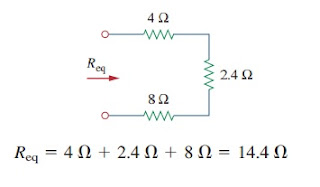# Parallel Resistors Circuit and Current Divider Simple Circuit

After discussing series resistors and voltage divider, let us learn about parallel resistors and current division. Parallel resistor is belong to one of the passive element.

## Parallel Resistors

Aside from series resistors, this type of circuit is the most common type of electric circuit.

For analyzing a parallel resistor circuit we can use the same method for the series resistor, using Kirchhoff’s laws and Ohm’s law.

Consider the circuit in Figure.(1),Figure 1. Parallel resistor connection

where two resistors are connected in parallel and therefore have the same voltage across them.

From Ohm’s law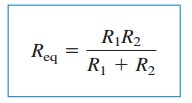(5)

Hence,

The equivalent resistance of two parallel resistors is equal to the product of their resistances divided by their sum.

Must be noted that the Equation.(5) only works for two resistors in parallel.

We can expand the Equation.(4) to the general case of a circuit with N resistors in parallel.

The equivalent resistance is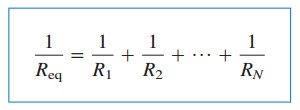(6)

Take a note that Req is always smaller than the resistance of the smallest resistor in the parallel connection. If R1 =R2 = … = RN = R, then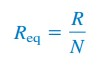(7)

For example, if 4 resistors with 100 Ω are connected in parallel, their equivalent resistance is 25 Ω.

It is easier to use conductance than resistance when dealing with resistors connected in parallel.

From Equation.(6) the equivalent conductance for N resistors is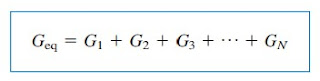(8)

where Geq = 1/Req,  G1 = 1/R1, G2 = 1/R2, G3 = 1/R3, GN = 1/RN

Equation.(8) states :

The equivalent conductance of resistors connected in parallel is the sum of their individual conductances.

It means we can redraw Figure.(1) with (2) where we replace the resistances to conductances.

The equivalent conductances of parallel resistors are obtained the same way as equivalent resistances of series resistors.

In opposite, the equivalent conductances of series resistors are obtained the same way as equivalent resistances of parallel resistors.Figure 2. Equivalent resistance or conductance

Hence the equivalent conductance Geq of N resistors in series is(9)

Given the total current i entering node a in Figure.(1) with the same values of voltage, we get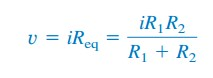(10)

## Current Divider

Combining Equations.(1) and (10) we get(11)

which shows the total current i is shared by the resistors in inverse proportion to their resistances.

This is known as the principal of current division, and the circuit in Figure.(1) is known as current divider.

Take a note that the larger current flows through the smaller resistance.Figure 3. Short and open circuit in parallel connection

Suppose one of the resistor in Figure.(1) is zero, say R2 = 0; so R2 is a short circuit, as can be seen in Figure.(3a).

From Equation.(11), R2 = 0 implies that i1 = 0i2 = i. This means that the entire current i bypasses R1 and flows through the short circuit R2 = 0, the path with least resistance.

When a circuit is short-circuited as can be seen in Figure.(3a), take note that :

1. The equivalent resistance Req = 0
2. The entire current flows through the short circuit.

For another extreme example where R2 = ∞ , that is, R2  is an open circuit as can be seen in Figure.(3b).

The current still flows through a path with least resistance, R1 .

Equation.(11) becomes(12)

In general, if a current divider has N conductors in parallel with source current i, the nth conductor will have current(13)

It is very convenient to combine resistors in series and parallel into single equivalent resistance Req.

Such equivalent resistance must have the same values of current and voltage as the original network at the terminal.

## Parallel Resistor Example

Let us review the example below for a better understanding

Find Req for the circuit in Figure.(4)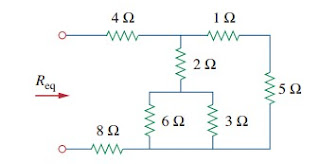Figure 4. Parallel connection example

6 Ω and 3 Ω in parallel

1 Ω and 5 Ω in series

2 Ω and 2 Ω in series

6 Ω and 4 Ω in parallel

Three resistors in series